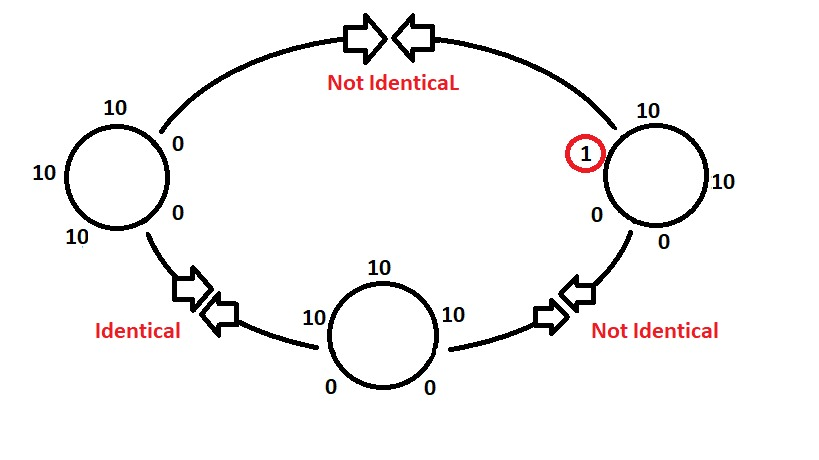# Python | Check whether two lists are circularly identical

Given two lists, check if they are circularly identical or not.Examples:

```Input : list1 = [10, 10, 0, 0, 10]
list2 = [10, 10, 10, 0, 0]
Output : Yes
Explanation: yes they are circularly identical as when we write the list1
last index to second last index, then we find it is circularly
same with list1
Input : list1 = [10, 10, 10, 0, 0]
list2 = [1, 10, 10, 0, 0]
Output :No
```

## Recommended: Please try your approach on {IDE} first, before moving on to the solution.

Method 1 : Using List Traversal

Using traversal, we have to double the given list. Check for any x(0<=n) to any x+n and compare with list2 to see if the list1 and list2 are same, if both are same then the list2 is circularly identical. Using two loops, check this property. The first loop will run from 0 to len(list1) and then check if the index (x to x+n) is same as list2, if yes then return true, else return false.

Below is the Python implementation of the above approach:

 `# python program to check if two  ` `# lists are circularly identical ` `# using traversal ` ` `  `# function to check circularly identical or not ` `def` `circularly_identical(list1, list2): ` `     `  `    ``# doubling list ` `    ``list3 ``=` `list1 ``*` `2` `     `  `    ``# traversal in twice of list1 ` `    ``for` `x ``in` `range``(``0``, ``len``(list1)): ` `        ``z ``=` `0`  `         `  `        ``# check if list2 == list1 curcularly  ` `        ``for` `y ``in` `range``(x, x ``+` `len``(list1)): ` `            ``if` `list2[z]``=``=` `list3[y]: ` `                ``z``+``=` `1` `            ``else``: ` `                ``break` `             `  `        ``# if all n elements are same circularly  ` `        ``if` `z ``=``=` `len``(list1): ` `            ``return` `True`  `     `  `    ``return` `False` `         `  ` `  ` `  `# driver code ` `list1 ``=` `[``10``, ``10``, ``0``, ``0``, ``10``] ` `list2 ``=` `[``10``, ``10``, ``10``, ``0``, ``0``] ` `list3 ``=` `[``1``, ``10``, ``10``, ``0``, ``0``] ` ` `  ` `  `# check for list 1 and list 2  ` `if``(circularly_identical(list1, list2)): ` `    ``print``"Yes"`  `else``: ` `    ``print``"No"` ` `  `# check for list 2 and list 3  ` `if``(circularly_identical(list2, list3)): ` `    ``print` `"Yes"`  `else``: ` `    ``print``"No"`

Output:

```Yes
No
```

Method 1 : Using map() function

Using Python’s inbuilt function map() we can do this in one single step, where we have to map list2 in a string and then see if it exists in the mapping of twice of list1 (2*list1) in another string.

Below is the Python implementation of the above approach:

 `# python program to check if two  ` `# lists are circularly identical ` `# using map function  ` ` `  `# function to check circularly identical or not ` `def` `circularly_identical(list1, list2): ` `    `  `    ``return``(``' '``.join(``map``(``str``, list2)) ``in` `' '``.join(``map``(``str``, list1 ``*` `2``))) ` `     `  ` `  `# driver code ` `list1 ``=` `[``10``, ``10``, ``0``, ``0``, ``10``] ` `list2 ``=` `[``10``, ``10``, ``10``, ``0``, ``0``] ` `list3 ``=` `[``1``, ``10``, ``10``, ``0``, ``0``] ` ` `  ` `  `# check for list 1 and list 2  ` `if``(circularly_identical(list1, list2)): ` `    ``print``"Yes"`  `else``: ` `    ``print``"No"` ` `  `# check for list 2 and list 3  ` `if``(circularly_identical(list2, list3)): ` `    ``print` `"Yes"`  `else``: ` `    ``print``"No"`

Output:

```Yes
No
```

My Personal Notes arrow_drop_upCheck out this Author's contributed articles.

If you like GeeksforGeeks and would like to contribute, you can also write an article using contribute.geeksforgeeks.org or mail your article to contribute@geeksforgeeks.org. See your article appearing on the GeeksforGeeks main page and help other Geeks.

Please Improve this article if you find anything incorrect by clicking on the "Improve Article" button below.

Article Tags :
Practice Tags :

Be the First to upvote.

Please write to us at contribute@geeksforgeeks.org to report any issue with the above content.